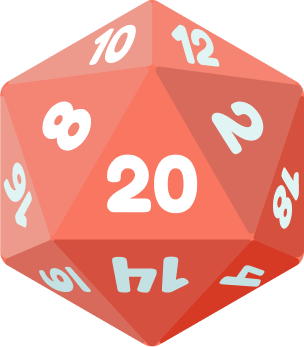### Random Variables & Distributions

A random variable quantifies chance events, and its probability distribution assigns a likelihood to each of its values.

The distribution contains all the information we have about the random variable, so it is a very important concept in probability theory.

In this quiz, we'll start with distributions of discrete random variables and then move on to the continuous case.

# Distributions

Roll a die in the shape of an icosahedron, a solid figure having twenty faces that are equilateral triangles.An icosahedron die

Let $X$ be the random variable that assigns a die roll its value; the range of $X$ is equal to the set $\{ 1, 2, \dots, 19, 20 \}.$ If the die is fair, what is $P(X = n) ?$

# Distributions

The probability distribution of the icosahedron die is called uniform because all of the likelihoods for the possible die rolls are the same.

There are plenty of nonuniform examples. Consider flipping a fair coin ten times in a row. Let $N$ be the number of heads in such a flip sequence.

Find a formula for $P(N = n)$ for $n = 0, 1, 2 , \dots, 10,$ the random variable's possible values.

Hint: The number of ways to choose $k$ objects from a set of $n$ is ${n \choose k} = \frac{n!}{k!(n-k)!},$ where $n! = n(n-1)(n-2) \cdots 1$ for $n \geq 1$ and $0! = 1.$

# Distributions

Any trial or experiment whose outcome can be classified as either a success or a failure is called a Bernoulli trial.

If the probability of success is $p,$ the probability of failure is $1-p,$ and when $T$ consecutive trials are performed, the number of successes $N$ is distributed as $P(N=n) = {T \choose n} p^{n} (1-p)^{T-n};$ this distribution for $N$ is called binomial because of the binomial coefficient ${T \choose n}.$

The coin flip is an example with $p = \frac{1}{2}, T = 10$; we'll see more examples later in the course.

# Distributions

Once we have $X$'s probability distribution, we can compute the expected value $E[X],$ which is the sum of all of $X$'s values weighted by their probabilities: $E[X] = \sum\limits_{n \in X\text{'s range}} n P(X = n).$ Let's say an unfair coin comes up heads three times out of every four flips. We flip this coin twice in a row; if $N$ is the number of times it lands heads up, $N$'s probability distribution is $P(N = n ) = {2 \choose n}\left( \frac{3}{4} \right)^{n} \left( \frac{1}{4} \right)^{2-n}.$ What's the average number of heads we should expect to see if we perform this coin flip experiment many times?

Good to Know: $\sum\limits_{j=0}^{n} a_{j}$ means $a_{0} + a_{1} + \dots + a_{n}$ and ${2 \choose 0} = 1, \ {2 \choose 1 } = 2 \ , {2 \choose 2 } = 1.$

# Distributions

Another valuable quantity we can find from $X$'s distribution is its variance \begin{aligned} \text{Var}[X]& = E[(X-E[X])^2] \\ & = E[X^2- 2 E[X] X +(E[X])^2] \\ & = E[X^2]-2 (E[X])^2+(E[X])^2 \\ & = E\big[X^2\big] - \big( E[X]\big)^2,\end{aligned} which measures the spread of observed values away from the expected one.

(These expected value properties will be explained in greater detail later in the course.)

What's the spread of the double-flip coin experiment from the last problem? Remember, if $N$ is the number of heads, $P(N = n ) = {2 \choose n}\left( \frac{3}{4} \right)^{n} \left( \frac{1}{4} \right)^{2-n}$ where ${2 \choose 0} = 1, \ {2 \choose 1 } = 2 \ , {2 \choose 2 } = 1.$

# Distributions

We find it useful to assign distribution functions to continuous random variables as well: $P( a \leq X \leq b) = \int\limits_{x = a }^{x=b} f(x) dx$ for some density function $f(x).$ The integral means the area below $f$'s graph and above the $x$-axis between $a$ and $b.$ In a loose sense, we think of $\int$ as a sum of continuous values, much like $\sum$ is a sum of discrete ones.

For instance, if the sample space is a fixed interval, say $[-2,3],$ we can choose $f(x)$ to be a constant; this choice gives us the uniform distribution.

What constant value must $f$ have in order for this density to define a probability distribution over the sample space $[-2,3] ?$

Hint: In each random experiment, it's certainly the case that we draw at least some $x$ from the sample space.

# Distributions

The density function allows us to find interesting characteristics of a continuous random variable, like its expected value: $E[X] = \int\limits_{\text{range of } X} x f(x)\, dx,$ which is very similar to the version for discrete random variables.

What is the expected value of the uniform distribution $f = \frac{1}{5}$ on $\Omega = [-2,3] ?$

Hint: If you want to avoid using calculus, remember that the graph of $\frac{x}{5}$ is a straight line through the origin, so $E[X]$ is related to the area of two triangles, one on either side of $x= 0.$

# Distributions

What is the variance of the uniform distribution on $[ -2,3] ?$ Recall that $\text{Var}[X] = E\big[X^2\big] - \big( E[X]\big)^2.$

Good to Know: If you want to avoid calculus, the area below the parabola $y=x^2$ and above the $x$-axis between $a$ and $b$ is $\frac{1}{3} [b^3-a^3].$

# Distributions

Discrete and continuous random variables and probability distributions are the central characters in our course.

We introduced these ideas in this quiz and the last; in the next quiz, we'll review by applying them to a few interesting real-world problems.

# Distributions

×